1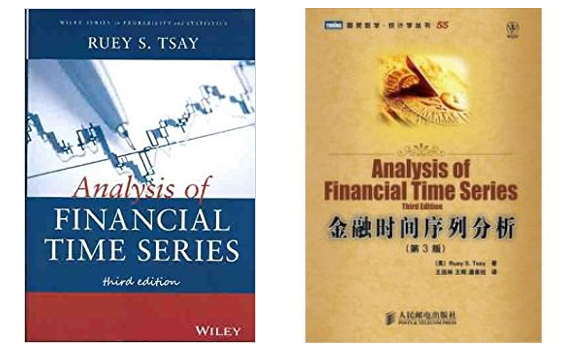2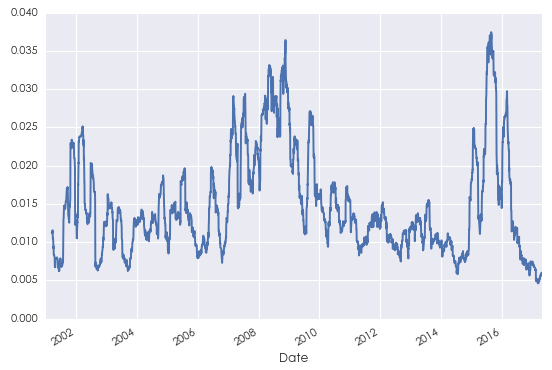3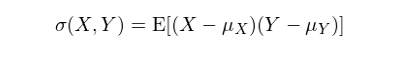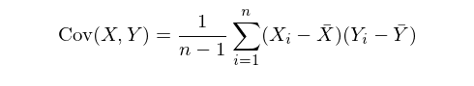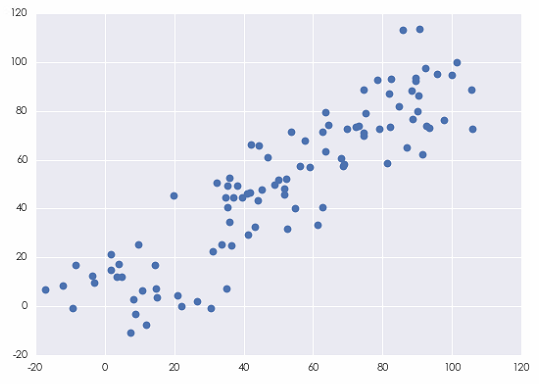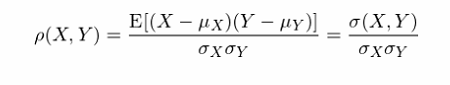ρ(X, Y) = 1 表示 X 和 Y 之间存在确切的线性正相关；

ρ(X, Y) = 0 表示 X 和 Y 之间不存在任何线性相关性；

ρ(X, Y) = -1 表示 X 和 Y 之间存在确切的线性负相关。4

1. 对于所有的时刻 t，有 E[r_t] = μ，其中 μ 是一个常数。

2. 对于所有的时刻 t 和任意的间隔 k，r_t 和 r_(t-k) 的协方差 σ(r_t, r_(t-k)) = γ_k，其中 γ_k 与时间 t 无关，它仅仅依赖于间隔 k。特别的，当 k = 0 时，这个特性意味着 σ(r_t, r_t) —— r_t 的方差——不随时间变化，等于一个与时间 t 无关的常数 γ_0，这称为方差平稳性（stationary in variance）

5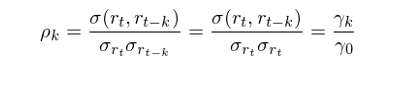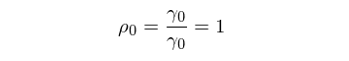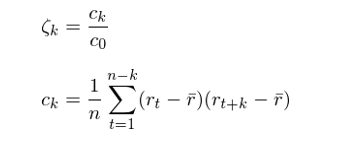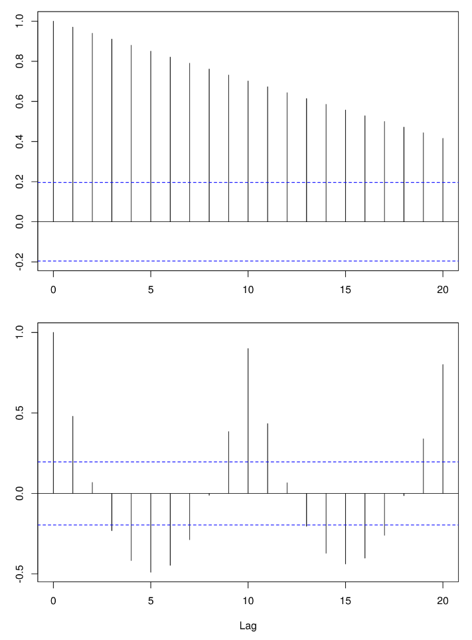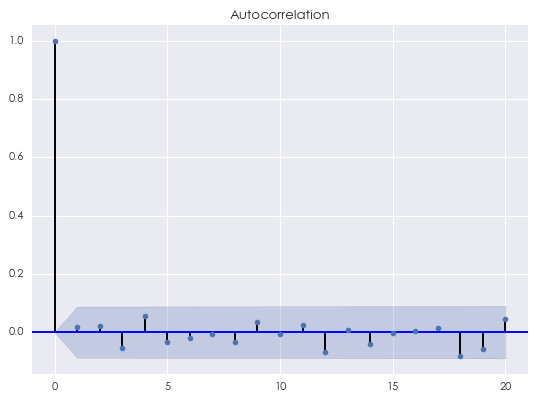1. 显然，间隔为 0 的自相关系数为 1；

2. 对于任意的 k ≥ 1，蓝色的阴影区域为 95% 的置信区间。因此，自相关系数只要没有超过蓝色阴影区域，我们就无法在 5% 的显著性水平下拒绝原假设（原假设为间隔为 k 的自相关系数为 0）。上图的结果说明当 k 不为 0 时，随机噪声的自相关系数为 0。

6

（全文完）